## Keynote Address 1stInternational Biophotonics Meeting in Israel Dec 9-11, 2012, Tel Aviv University

#### slide 1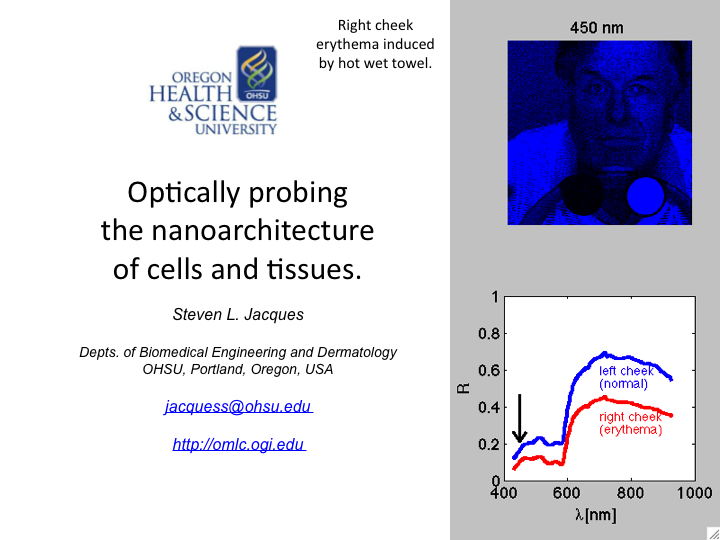1. This talk discusses how light can probe the nanoarchitecture of cells and tissues. The emphasis is on the process of light scattering by tissue structures both larger than and smaller than the wavelength of light.

#### slide 2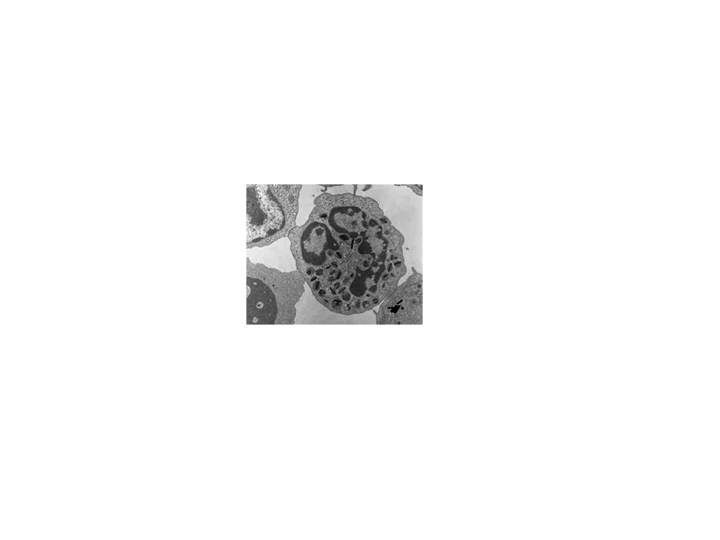2. This is an electron microscope image of a cell. Note the variety of sub-cellular structures which are on the scale of a wavelength of light. Such structures efficiently scatter light.

#### slide 3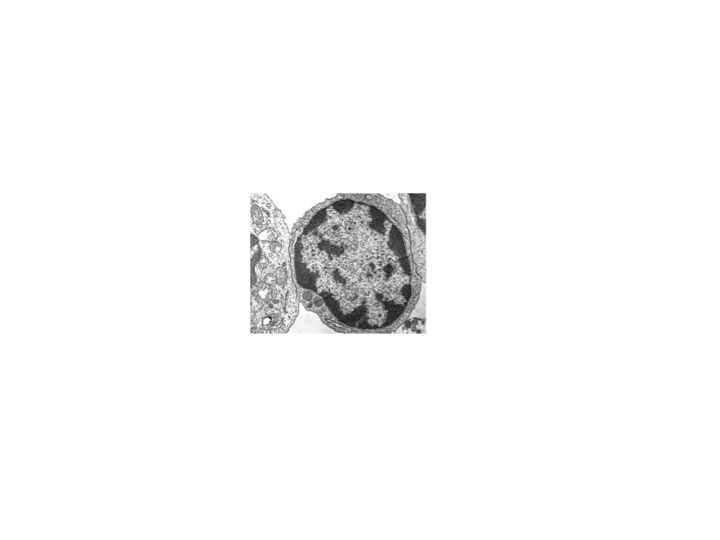3. This is another EM image of a cell.

#### slide 4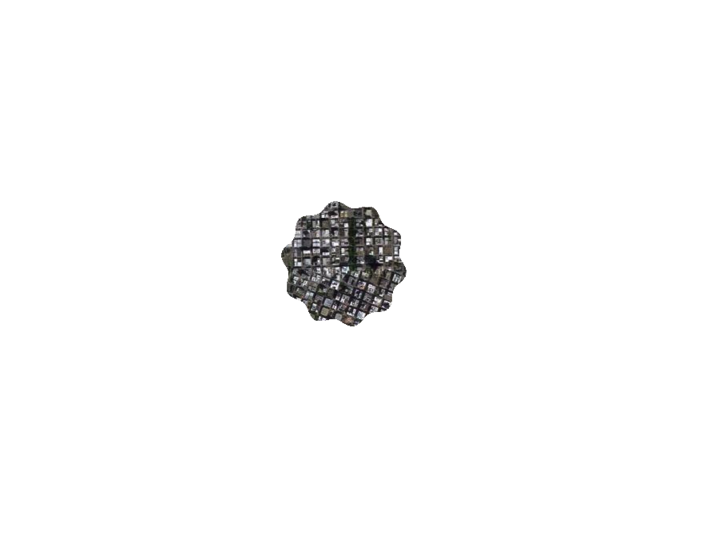4. This is another image... not of a cell but ...

#### slide 5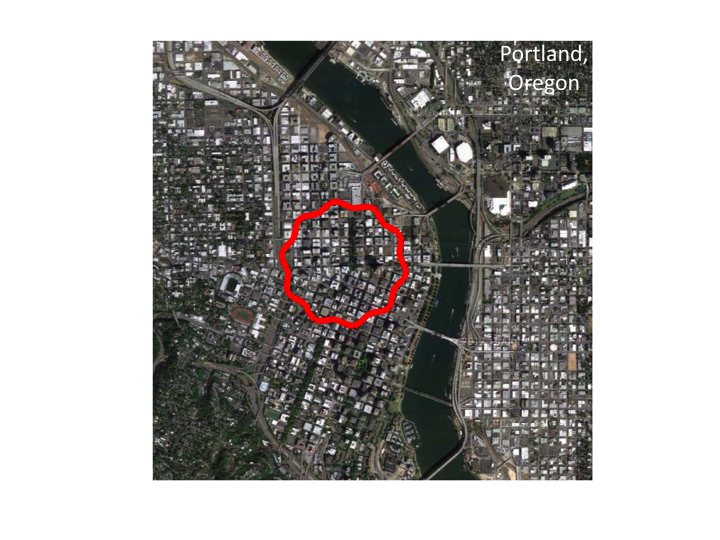5. ...an image of Portland, Oregon, my home town, as viewed from an airplane. Note that the distribution of sizes of structures are similar to the distribution seen in cells. The size distribution is fractal.

#### slide 6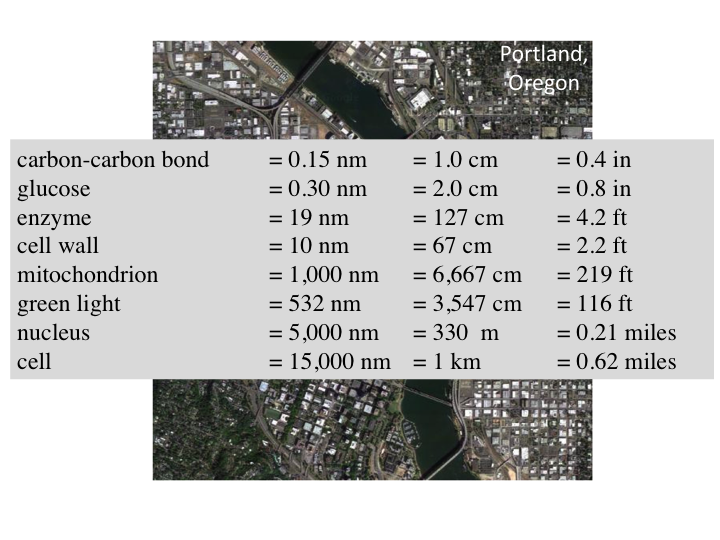6. On this scale, a carbon-carbon is about 1 cm, glucose fits in the palm of my hand, and other measures are shown. A cell is like the downtown area of a city. Green light passing through a cell is like an ocean wave moving through the city, scattering off cars, buildings, trees and other structures. From outside the city, optical measurements can observe the scattered waves and deduce information about the size distribution of structures within the city/cell that caused the scattering.

#### slide 7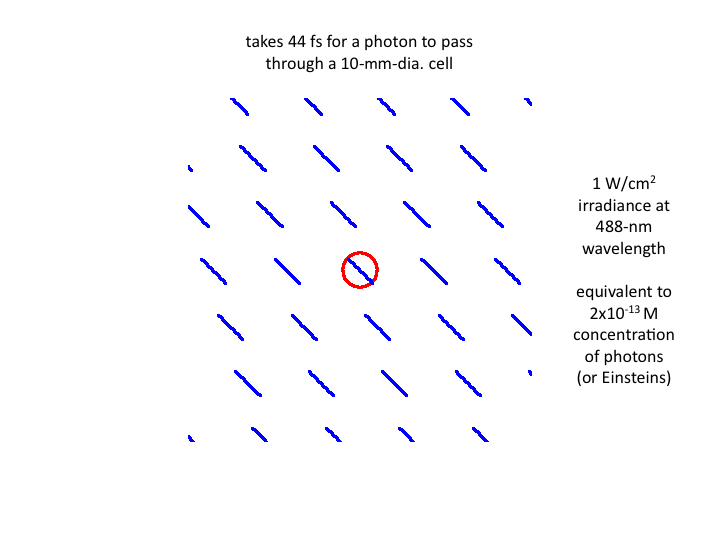7. Waves of blue light at 1 W/cm2 pass through a cell in waves, with each wave taking 44 fs to transit the cell. The equivalent number density of photons is 2x10-13 moles/liter (or Einsteins).

#### slide 88. Returning to our view of the city/cell, let us move further outward so the cell looks smaller.

#### slide 9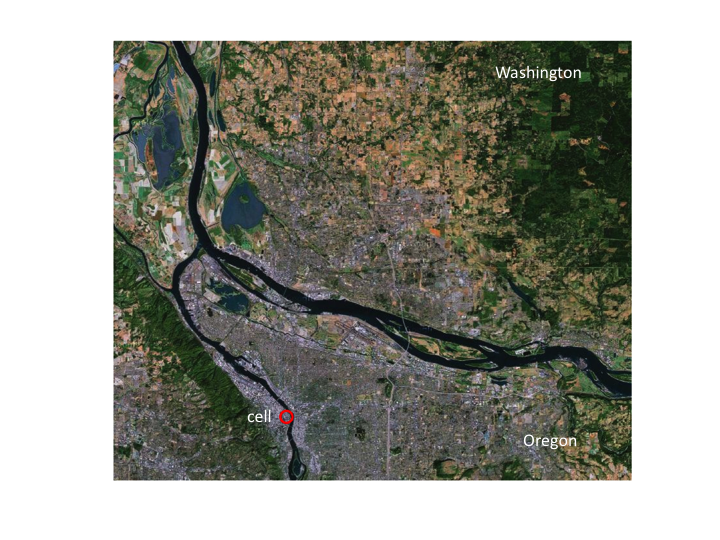9. Now the cell is a very small part of a larger landscape. In this picture, the state of Washington is to the north of the Columbia River and Oregon is to the south of the river.

#### slide 10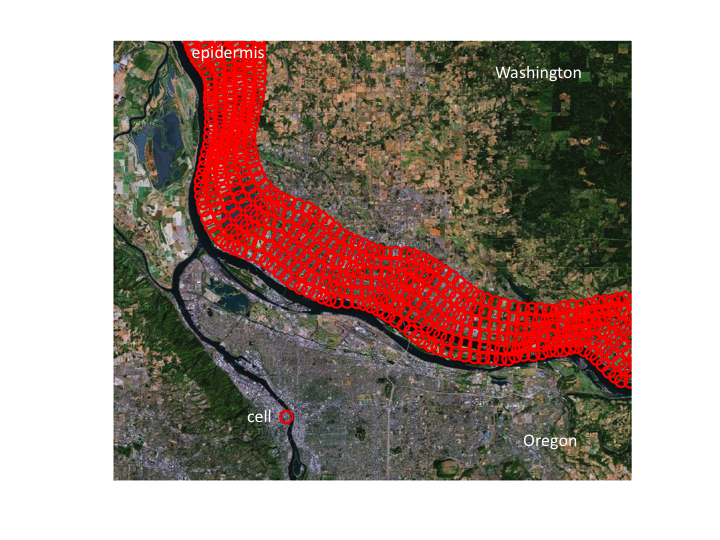10. An epidermis would appear this thick on this scale.

#### slide 11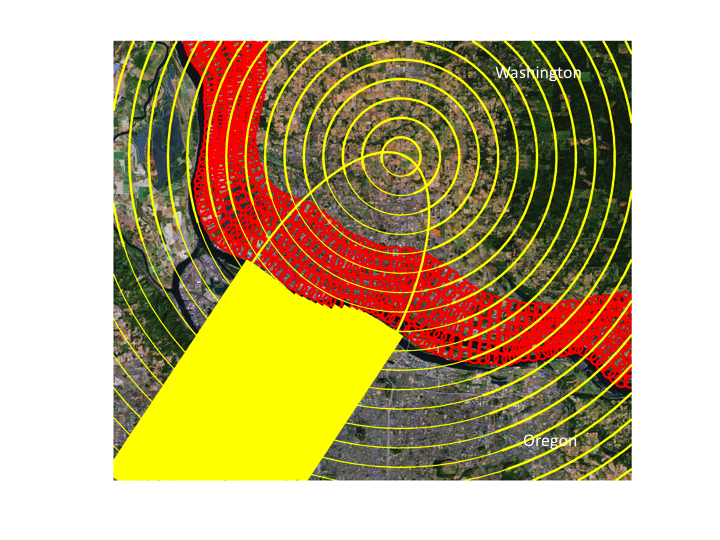11. If a laser was directed from Oregon into Washington, it would penetrate through the epidermis into the dermis. It would be possible to focus the light within the epidermis and the superficial dermis, but by the time the light reached a depth of 1 transport mean free path (mfp' = 1/(μs(1-g)). shown as the center of circles of diffusion), the light is sufficiently scattered that it can no longer be focused. Photons now move down photon concentration gradients.

#### slide 12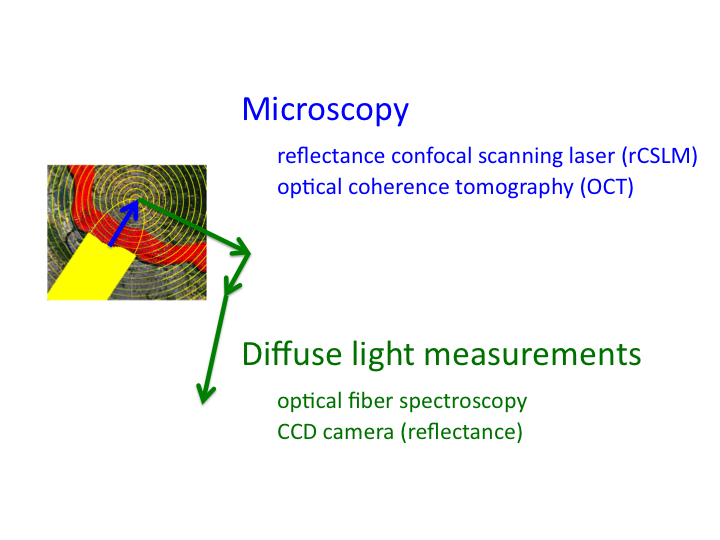12. Hence, the region with the blue arrow is the domain of microscopy, while the domain of the green arrows is the domain of diffuse light measurements.

#### slide 13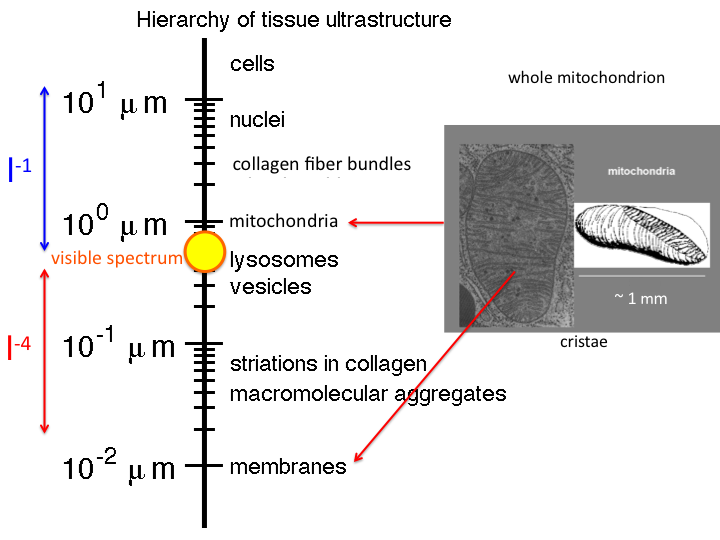13. There is a hierarchy of tissue ultrastructure, ranging from membranes (10 nm) to cells (>10 μm). The range of visible light is shown by the yellow circle. We can easily measure light from about 300 - 1300 nm. Many structures are much bigger than this range of wavelengths, and scattering by such structures is called "Mie Scattering", which falls as approximately λ-1. Also many structures are much smaller than the wavelengths of visible light, and scattering by such structures is called "Rayleigh Scattering", which falls as λ-4. Structures like mitochondria present both large and small structure, and hence contribute both Mie and Rayleigh scattering.

#### slide 14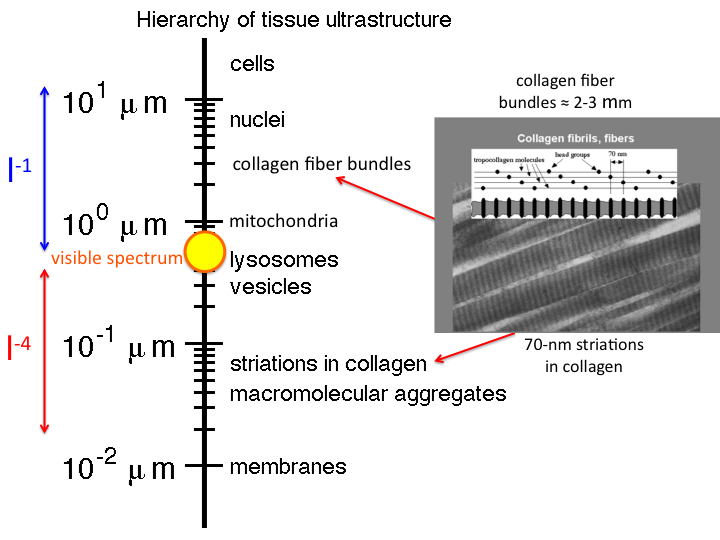14. Similarly, collagen fibers present both Mie and Rayleigh scattering.

#### slide 15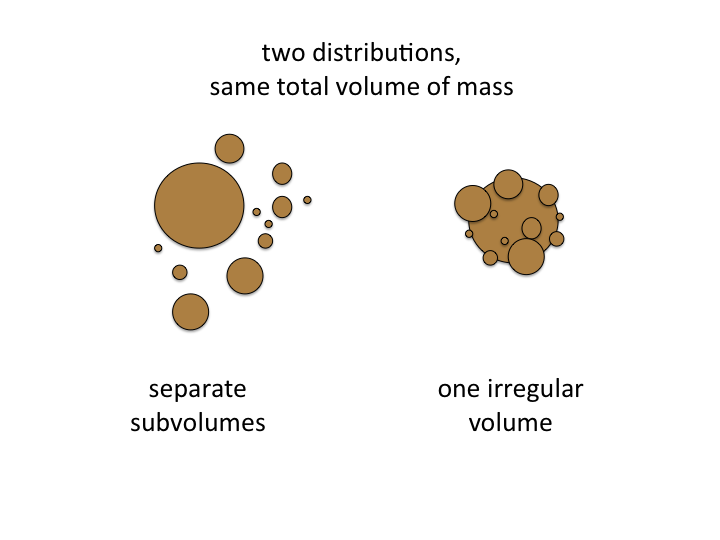15. In general, cells and tissue present structures which are complex is shape and are irregularly aggregated. To model the true biological structure is not possible, but we can "mimic" the optical scattering by an equivalent "Mie Model" in which a collection of spheres of different sizes at particular concentrations recreates the optical behavior of the tissue. Such a "mimic" is a useful metric for characterizing a tissue.

#### slide 16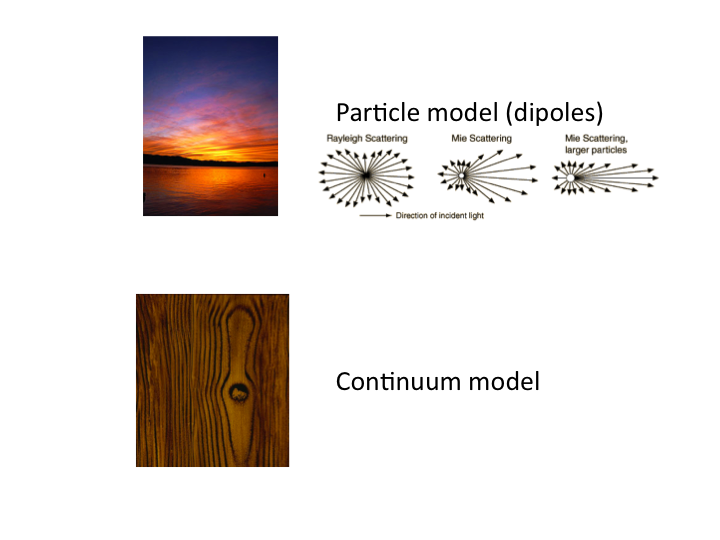16. There are two approaches toward modeling tissue scattering: (1) the Mie theory model, which is commonly understood in terms of the blue sky and red sunset, and (2) the continuum model, which describes the spatial distribution of refractive index fluctuations, like the grains of wood.

#### slide 17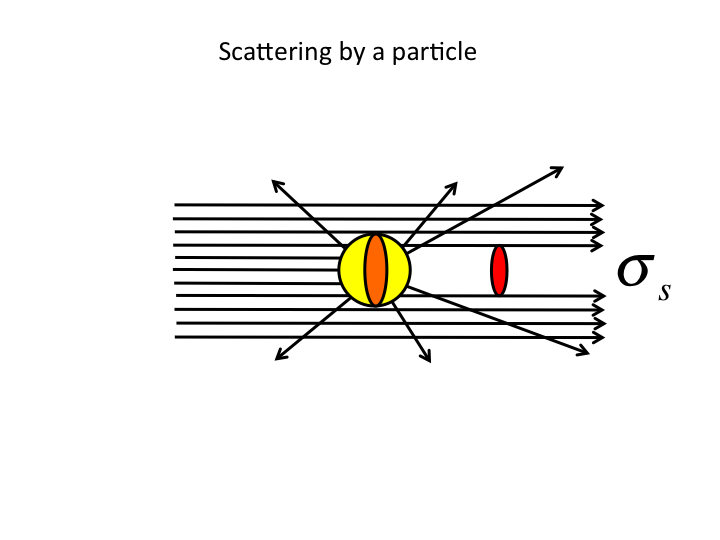17. First, some definitions of optical scattering properties. The scattering cross-sectional area, σ [µm2], acts like a shadow cast by a light-scattering particle.

#### slide 18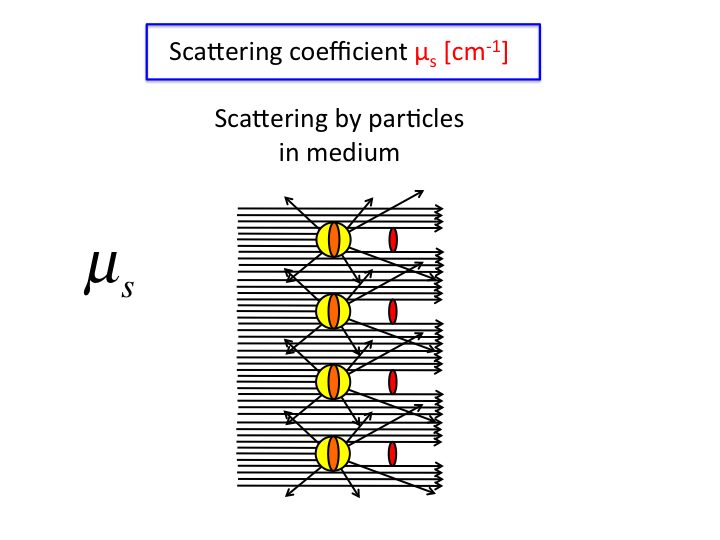18. Multiplying σ [µm2] by the number density, ρ [#/µm3], yields the scattering coefficient, µs-1]. Collimated transmission through a cuvette of length L [µm] holding a solution of µs is T = exp(-µsL).

#### slide 19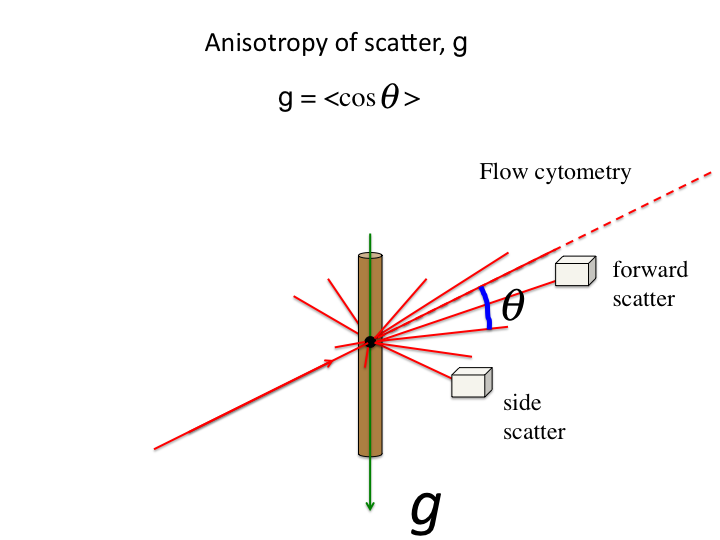19. When a scattering event occurs, the photon is deflected by an angle θ. The anisotropy, g [dimensionless], is the average value of cos(θ).

#### slide 20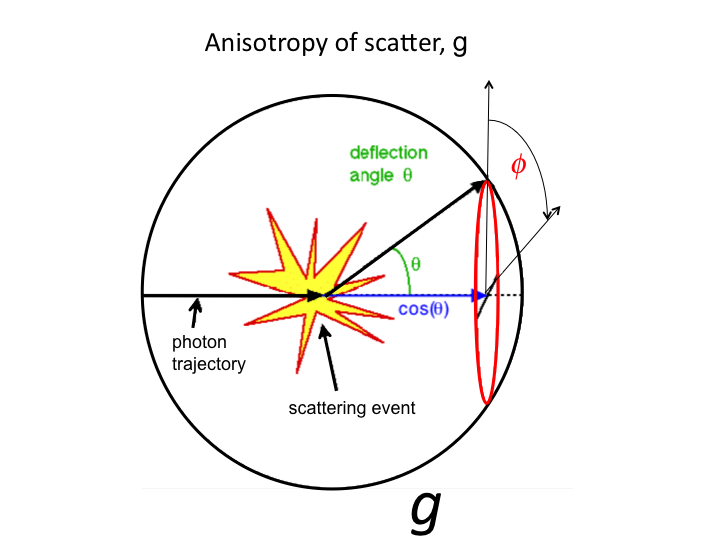20. The cos(θ) is the projection (blue line) onto the original axis of photon propagation. Light scatters both forward and backward. The average value g = <cos(θ)> characterizes the effectiveness of scattering. As g approaches 1.0, the light is so very forward scattered that scattering is not so effective.

#### slide 21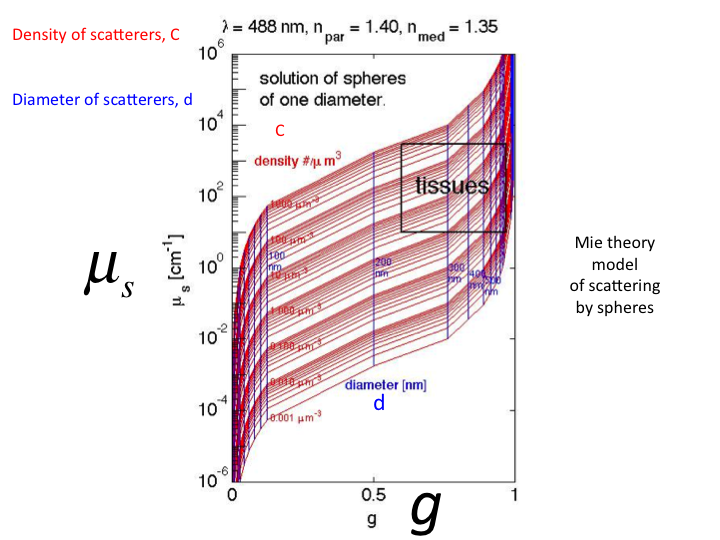21. If we use Mie Theory to plot all possible values of µs versus g using a single size sphere, we get this set of curves. The red contours show increasing density ρ [#/µm3]. The vertical blue lines show increasing sphere diameter [µm]. (The µs is here shown as [cm-1].) Typical values for cells and tissues lay in the black box in the upper right of the figure.

#### slide 22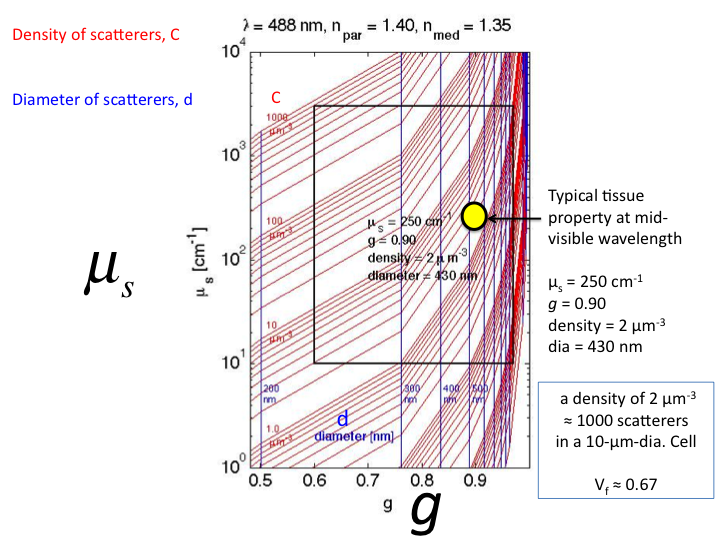22. This is a close-up of the region were tissue properties lay. The yellow circle is a typical tissue value in the mid-visible wavelength range. In other words, a solution of microspheres of diameter 430 nm and refractive index 1.40 at a concentration of 2 [µm3] within a solution of average refractive index 1.35 would yield µs = 250 cm-1 and g = 0.90 at the wavelength λ = 488 nm (blue light), thereby mimicing the tissue properties. This concentration corresponds to a volume fraction (vf) of 0.67, at about 1000 scattering spheres per 10-µm-dia. cell.

#### slide 23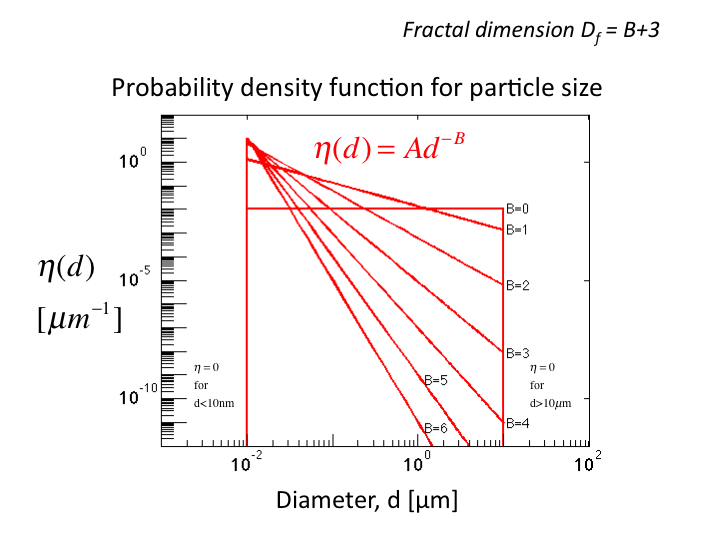23. Now consider a distribution of sphere sizes, η(d), where d is the sphere diameter [µm]. The distribution is characterized by a parameter B. (Often the fractal dimension is cited as Df = B+3.) The area under each curve is set to 1.0. Artificially, spheres below 10 nm or above 10 µm are excluded, since the contribution to scattering from such structures is minor. Scattering is dominated by structures closer to the wavelength of the light being scattered. As B increases, the curves become more sloped, having more small scale Rayeigh scattering and less larger scale Mie scattering.

#### slide 24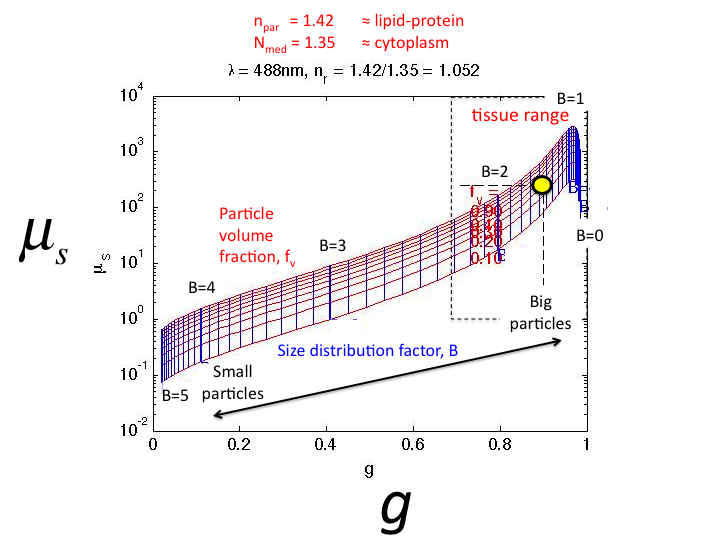24. Using Mie Theory to calculate the µs and g for solutions with varying B, the graph shown is generated. The red lines show constant particle volume fraction, fv = 0.1 to 0.9. The vertical blue lines show constant values of the size factor B = 0 to 5. Tissues sit in the black box in the upper right corner. As B decreases, both µs and g increase.

#### slide 25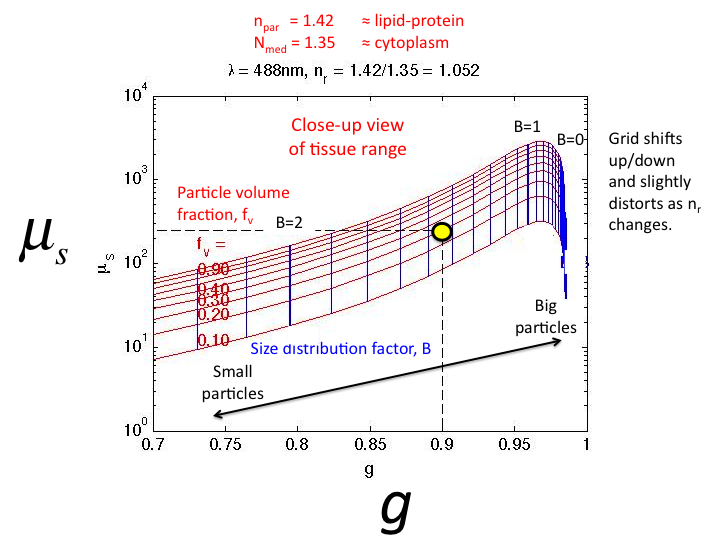25. A close-up of the tissue region of µs vs g. The typical tissue value (yellow circle) corresponds to fv = 0.30 (70 percent water) and B = 1.65.

#### slide 26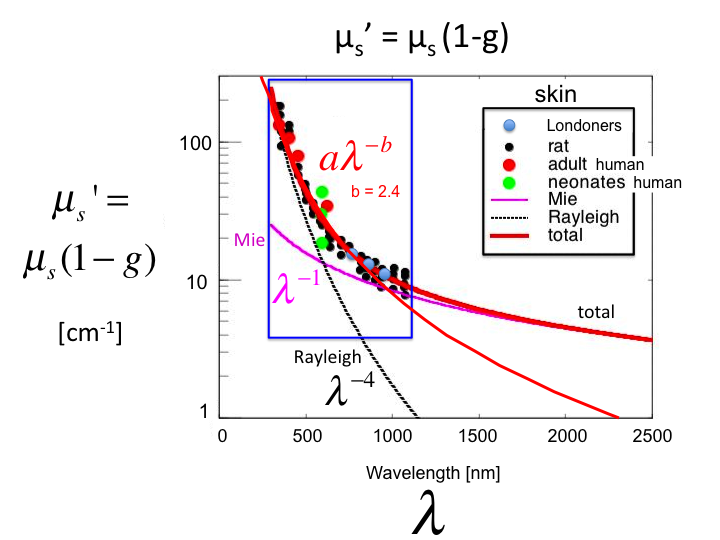26. A plot of the values of µs' = µs(1-g) [cm-1] versus wavelength λ [nm] for skin, based on experiments in humans and rats. The shorter λ behavior is dominated by Rayleigh scattering (λ-4], and the longer λ behavior is dominated by Mie scattering (λ-1]. However, in the region of practical optical measurements between 300-1300 nm, one can characterize the behavior by a single parameter b, such that µs' = aλ-b. In this case, the value of b for skin is 2.4.

#### slide 27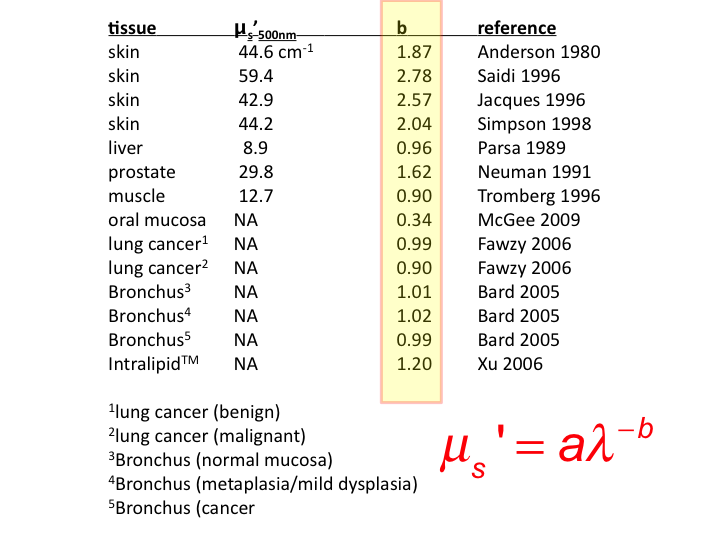27. The literature cites values of b for various soft tissues and for skin. Soft tissues show b close to 1.0, while skin values are

#### slide 28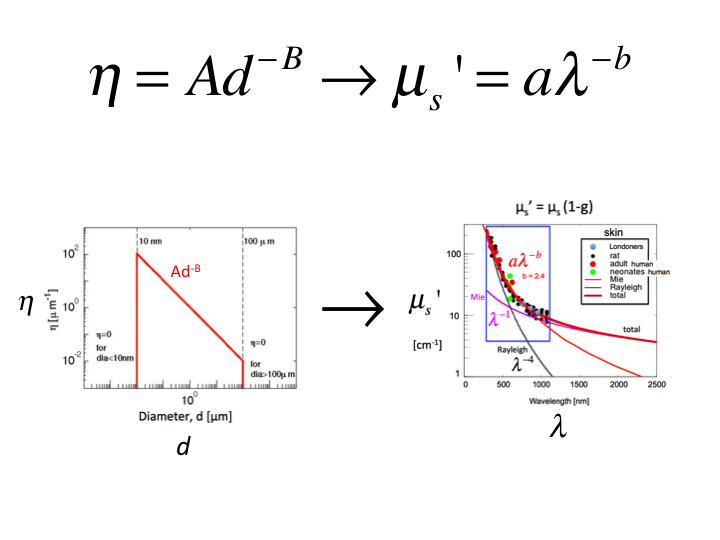28. Let us choose a range of B values to predict the properties of tissue with varying Rayeigh versus Mie scattering, and calculate using Mie Theory the optical properties versus wavelength, µs'(λ), then fit the data by aλ-b to yield a value b that characterizes the tissue. In this way, we generate the relationship between B and b.

#### slide 29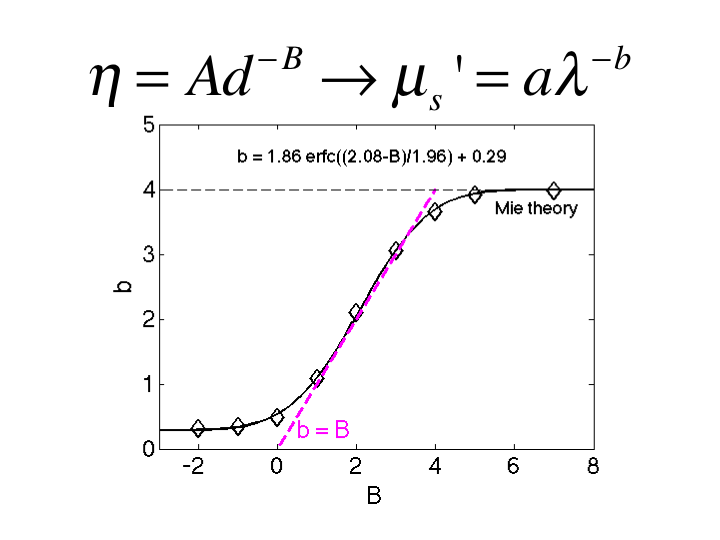29. This figure summarizes the relationship between B and b. As B increases to >6, the b approaches 4, consistent with Rayleigh scattering. As B drops toward 1, the b drops to 1, consistent with Mie scattering. In the region 1<B<3, the value of b equals the value of B. This is a convenient relationship appropriate for biological tissues.

#### slide 30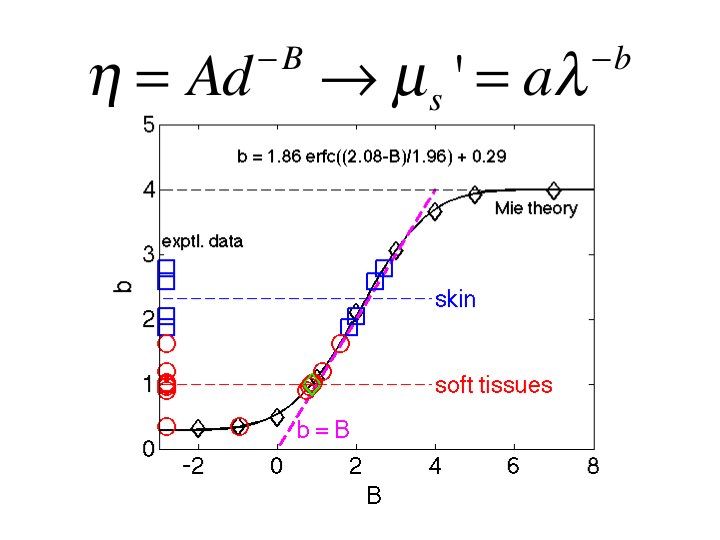30. Adding the data from the literature, the soft tissue show both b and B equal to 1.0 and equal to 2 or more for skin.

(NOTE: The description presented in this talk has not attempted to include the "packing factor" effect due to close packing of sphere, which generally lowers the scattering properties due to constructive interference of scattered waves from the spheres. The "packing factor" is an important complication that must be considered. But as a metric, the b and B descriptors are simply characterizing the "effective" size distribution of the tissue.)

#### slide 31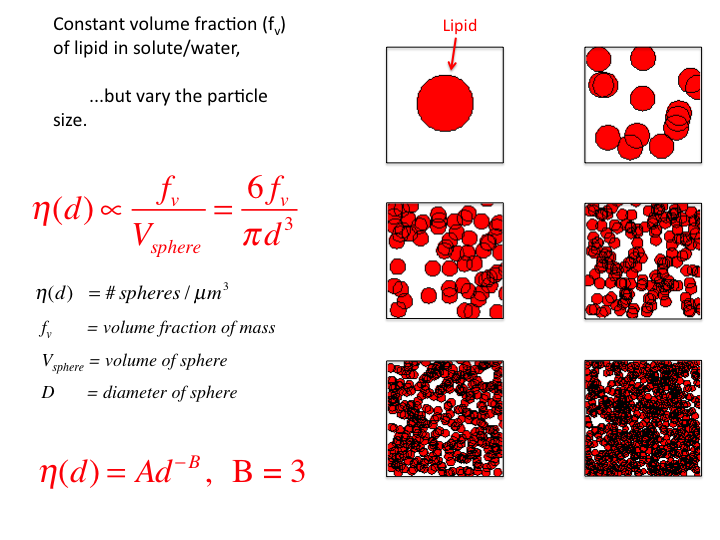31. Consider a tissue with a volume fraction of lipid fv = 0.10 (n = 1.46, within a salty proteinaceous medium of n = 1.35). Let the diameter (d) of the droplets vary while keeping the fv constant. As the size decreases, the number density increases to keep fv constant. Consequently, the size distribution η(d) varies as d-3, and B = 3.

#### slide 32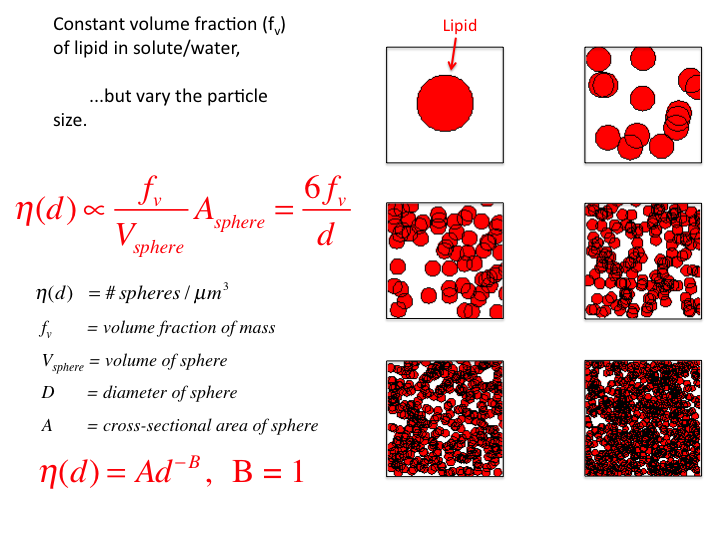32. If one scales this η(d) by the cross-sectional area of the spheres, Asphere, then the η(d) varies as d-1, and B = 1. This scaling is not justified by any physical rationale, but simply shows what is required to cause η(d) to vary as d-1.

#### slide 33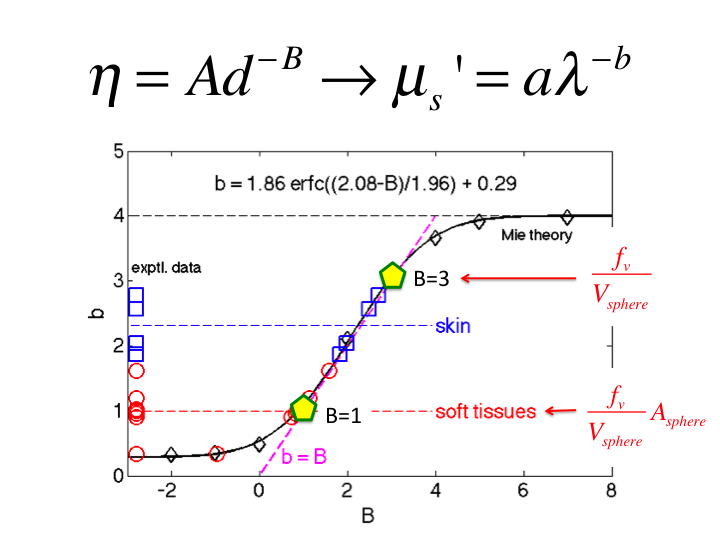33. Hence, the behavior of tissue falls between these to descriptions of η(d), scaling as d-3 and d-1.

#### slide 34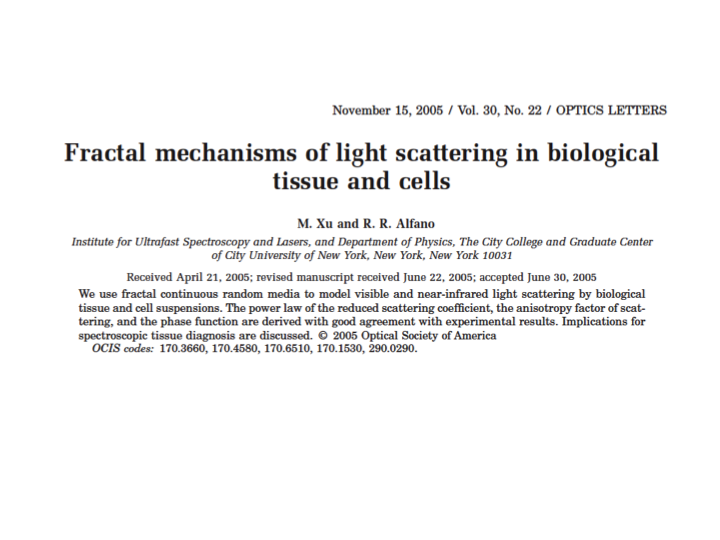34. The alternative description of light scattering is the continuum model for light scattering. This paper by Xu and Alfano (2005) was an early paper on this topic, and utilized the data from Schmitt and Kumar (1996) that described the distribution of spatial frequencies of density fluctuation in tissues. I will not attempt to summarize the continuum model, but I do note that it eventually predicts the aλ-b behavior of light scattering in tissues. Hence, both the Mie Theory and Continuum Theory eventually find their justification in matching experimental data.

#### slide 35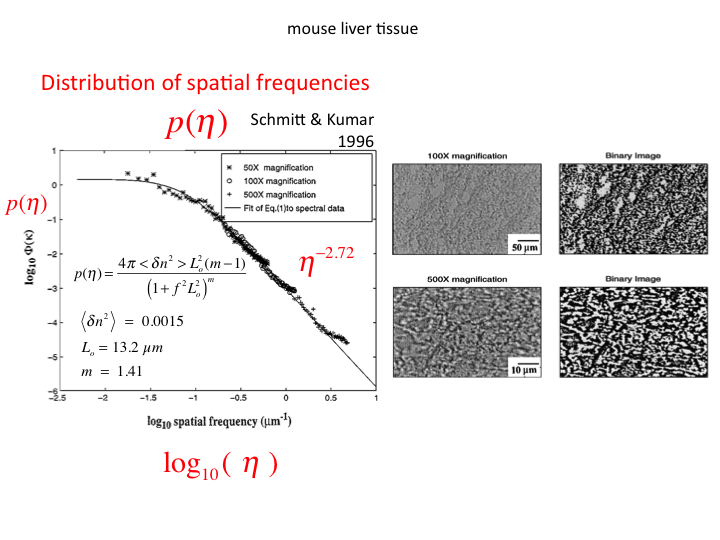35. Schmitt and Kumar specified the distribution of spatial frequencies in several tissues (figure shows liver).

#### slide 36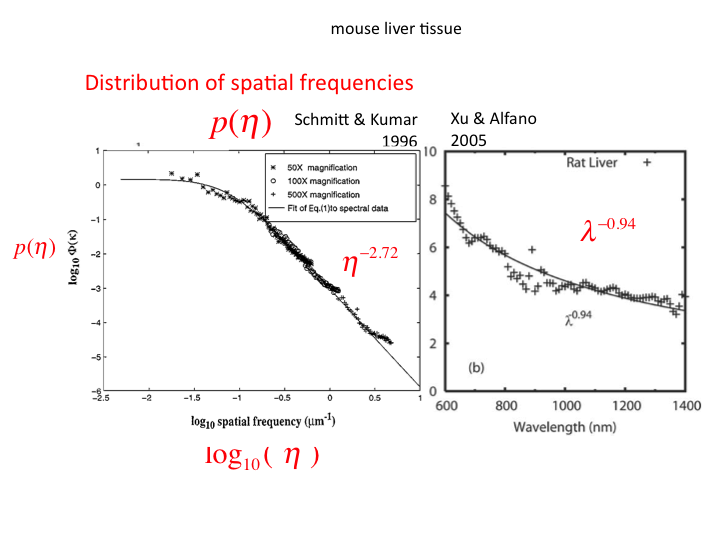36. Xu and Alfano mapped the distribution of spatial frequencies into an aλ-b behavior of light scattering in tissues.

#### slide 37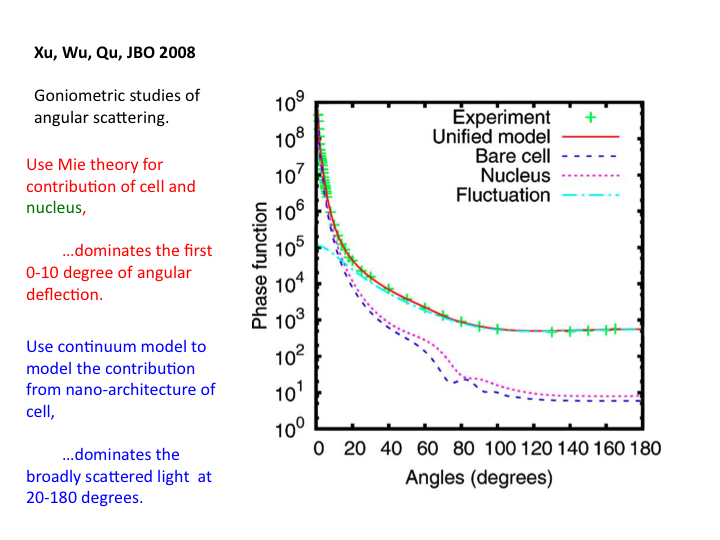37. A recent paper by Xu et al. (J Biomedical Optics 2008) is especially informative. It reports on the angular dependence of scattering, as compared to the Mie Theory prediction of scattering from a cell and a nucleus, and the Continuum Theory prediction of scattering from the sub-cellular nanoarchitecture, which dominates the scatter at angles broader than 20 degrees.

#### slide 38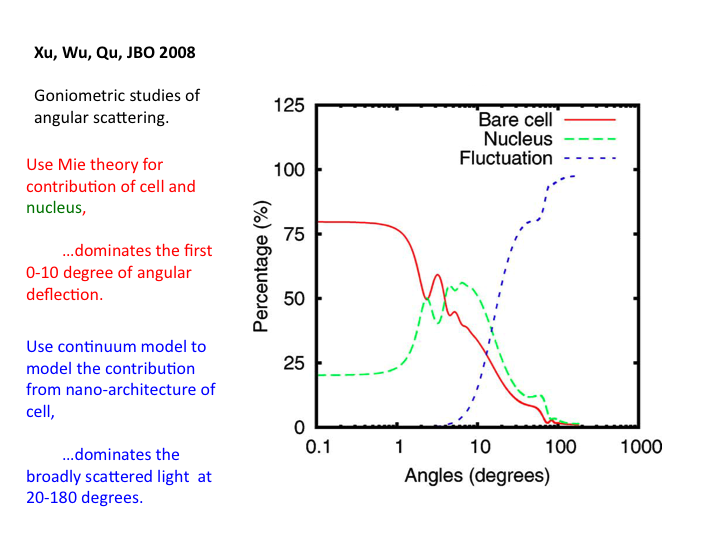38. The relative contributions to scatter from the bare cell, the nucleus and the nanoarchitecture are shown as a function of angle. Above 20 degrees the nanoarchitecture dominates the scattering.

#### slide 39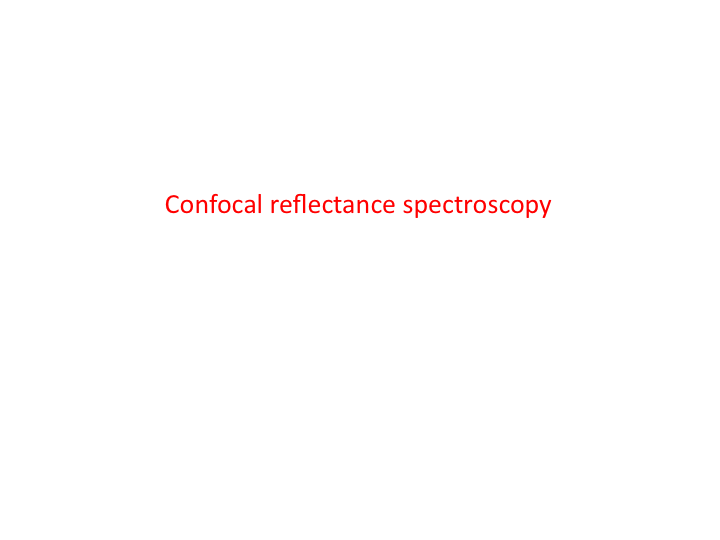39. A variety of measurements of optical scattering by tissues is possible. One example is confocal reflectance microscopy, which involves delivery and collection of light from a confocal volume of tissue.

#### slide 40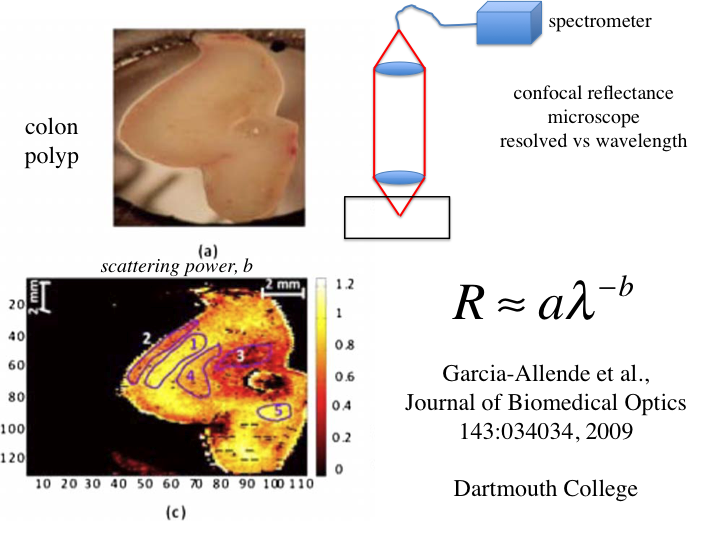40. A study by the group at Dartmouth College used a confocal reflectance spectrometer to yield reflectance versus wavelength, R = aλ-b. This is approximately similar to the story of µs' = aλ-b. A colon polyp is pressed against glass and an image taken, which does not show much structure. However, the image achieved by scanning the confocal spectrometer yielded an image based on b, which shows a lot of structure in the polyp. Hence, the scattering power b serves as a contrast parameter for imaging.

### Summary

In summary, optical measurements that measure the scattering coefficient, µs, and the anisotropy of scattering, g, can characterize the effective size distribution of the nanoarchitecture of tissues, as well as the contributions from the bare cell and the nucleus. Hence, non-invasive optical measurements can monitor ultrastructure changes in cells and tissues.